# 2D shapes

A 2D shape is a shape that lies in a plane and only has a length and a width, but no height or depth.

2D shapes can be classified as closed shapes and open shapes. A closed shape means its sides are connected from end to end with no break in the connection. Comparatively, an open shape means at least one endpoint of one side is not connected to the rest.

## Closed 2D shapes

Closed 2D shapes are studied extensively in geometry. The following are some important 2D shapes.

### Polygons

A polygon is a closed 2D figure formed by three or more non-collinear line segments, called sides. Polygons can be further classified based on their characteristics.

Polygons classified by number of sides. The table below listed some of them.

PolygonNo. of sidesFigure
Triangle 3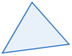Quadrilateral 4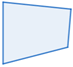Pentagon 5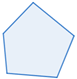Hexagon 6Polygons classified by shape

Regular polygonIrregular polygon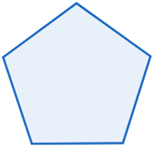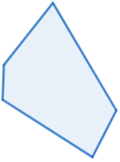The regular pentagon above has five congruent sides and five congruent interior angles. The irregular pentagon above has sides and interior angles that are not all congruent.

Convex polygonConcave polygon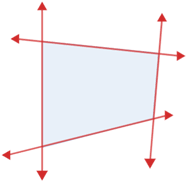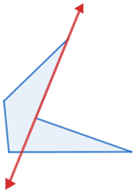A line containing any side of the polygon does not intersect the interior of a convex polygon. A least one line containing a side of a concave polygon intersects its interior.

### Circles

A circle is a 2D curve in which all of the points on the curve lie the same distance from a fixed point. The fixed point is called the center of the circle, and the distance from the center to any point on the circle is called the radius.

### Ellipse

An ellipse is a 2D curve in which all of the points on the curve lie the same total distance from two fixed points. The two fixed points are called the focal points. A circle is a special ellipse in which the two focal points overlap.

While a circle or a ellipse can informally be considered a polygon with infinitely many sides, it technically is not a polygon.